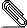# [NTG-context] section with \sethupead[section][alternative=text]; other type of section misbehaves..

```When using \setuphead[section][alternative=text] I noticed two things:

1. There is an extra space (horizontally) directly after the section
heading. I can't get a grip on how to get rid of it.
2. A different type of section (define with \definehead[summary][section]
seems to alter it's behaviour due to a \setuphead[section][..]. Shouldn't
the summary behaviour be independend of what one sets for section?```
```
Here is a MWE that shows the problem:

example 1:

\defineblank[sprong][.5cm]
\setupwhitespace[none]
\setupindenting[yes, small, next]
\definetextbackground
[SummaryBackground]
[frame=off,frameoffset=0pt,
background=color,
backgroundcolor=gray,
backgroundoffset=2ex]
\starttext
\section{First sentence. Second sentence.}\input zapf
\summary{Summary}\input zapf
\stoptext

example 2:

\defineblank[sprong][.5cm]
\setupwhitespace[none]
\setupindenting[yes, small, next]
\definetextbackground
[SummaryBackground]
[frame=off,frameoffset=0pt,
background=color,
backgroundcolor=gray,
backgroundoffset=2ex]
\starttext
\section{First sentence. Second sentence.}\input zapf
\summary{Summary}\input zapf
\stoptext

Adding [alternative=paragraph] for summary solves this problem, and is at
least a workaround, but that should not be necessary.

My main problem though is how to get rid of that extra horizontal space
after the normal section heading. The next sentence should align just like
the section is a normal sentence itself (only typeset as bold).

Is there a solution for that?

Many thanks.

Greeting,

Rob

-- attached (example 1 = test5a.pdf; example 2 = test5b.pdf)```test5a.pdftest5b.pdf
```___________________________________________________________________________________